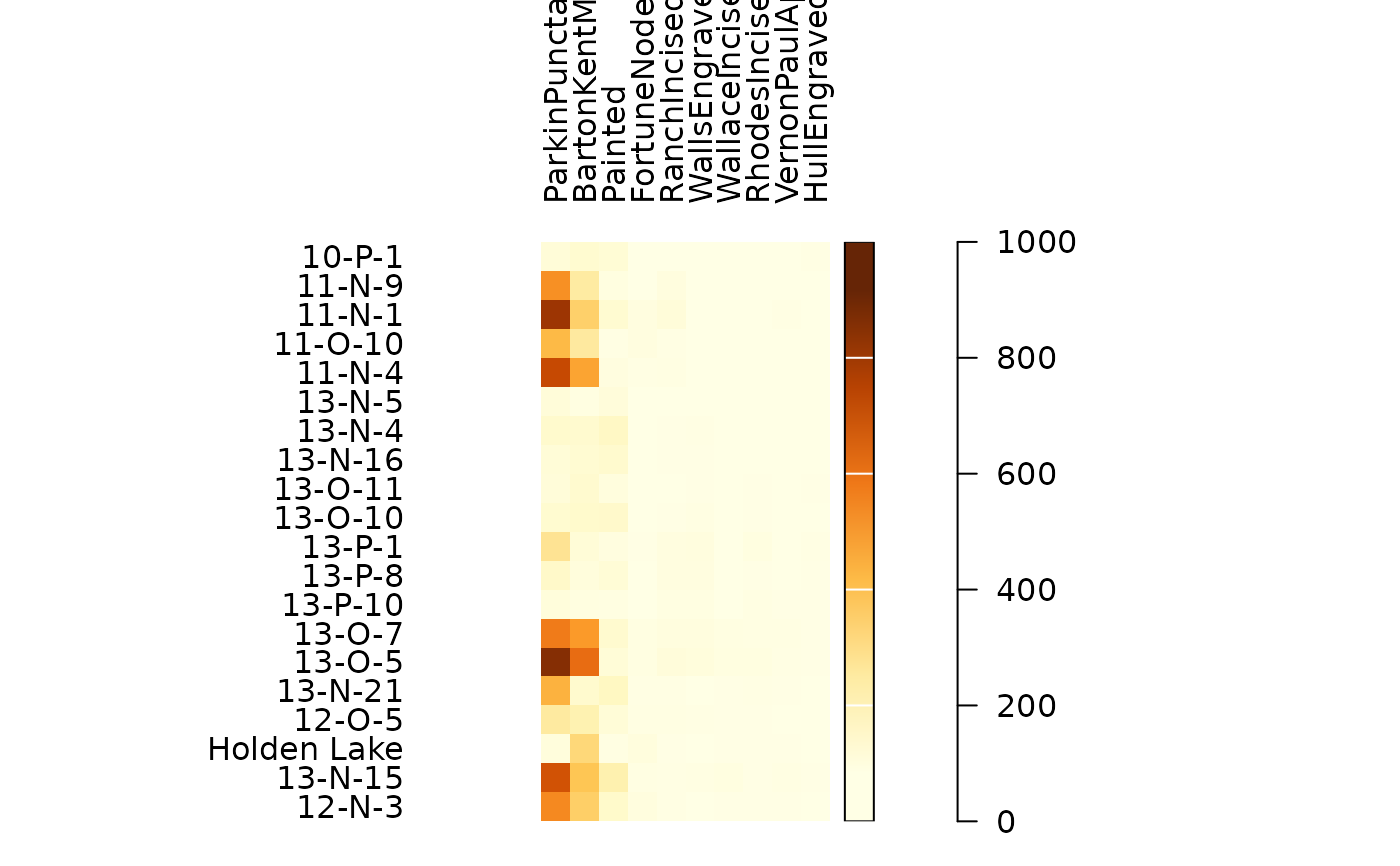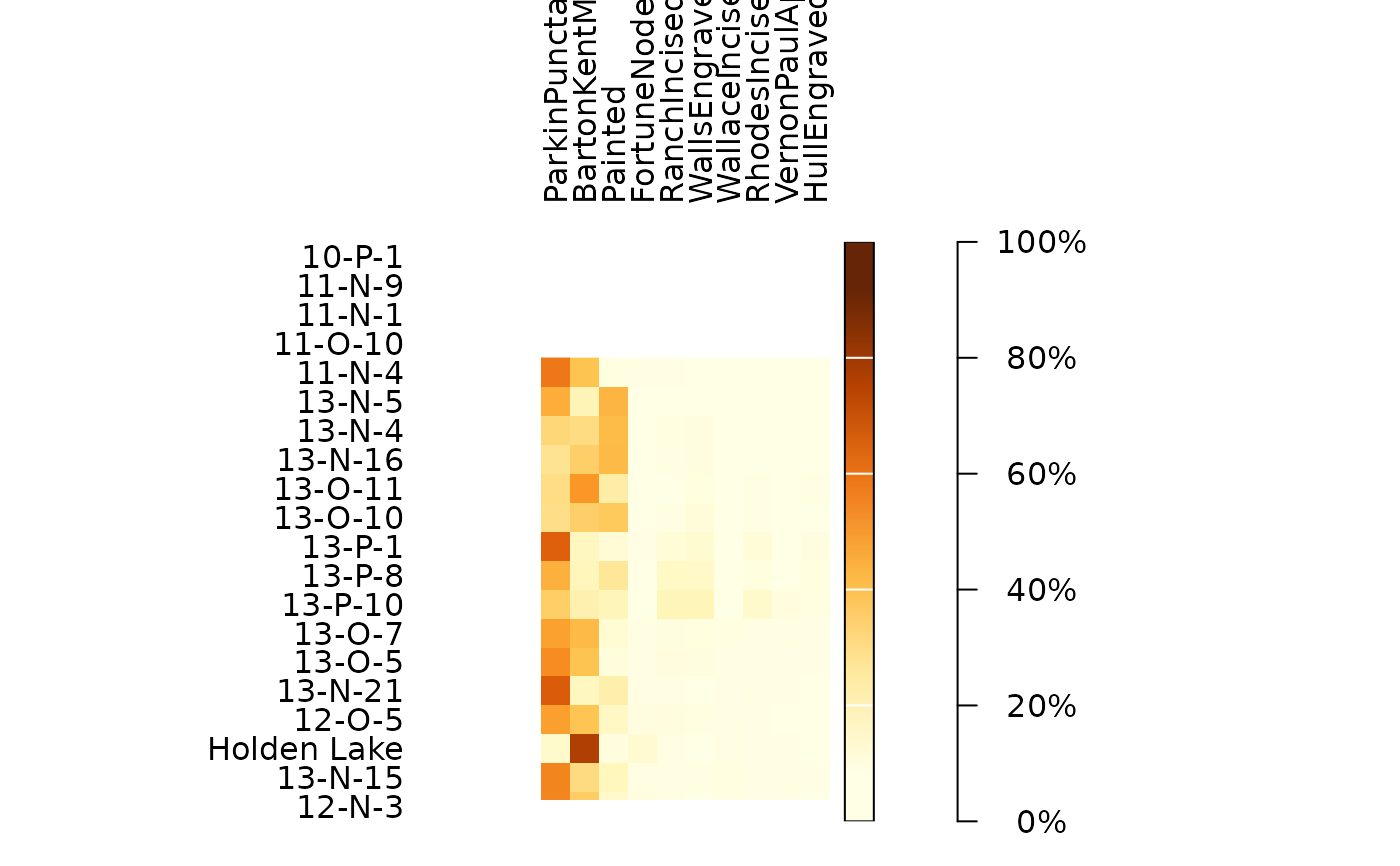Plots a heatmap.

## Usage

plot_heatmap(object, ...)

# S4 method for matrix
plot_heatmap(
object,
col = grDevices::hcl.colors(12, "YlOrBr", rev = TRUE),
diag = TRUE,
upper = TRUE,
lower = TRUE,
freq = FALSE,
margin = 1,
axes = TRUE,
legend = TRUE,
...
)

# S4 method for data.frame
plot_heatmap(
object,
col = grDevices::hcl.colors(12, "YlOrBr", rev = TRUE),
diag = TRUE,
upper = TRUE,
lower = TRUE,
freq = FALSE,
margin = 1,
axes = TRUE,
legend = TRUE,
...
)

# S4 method for dist
plot_heatmap(
object,
col = grDevices::hcl.colors(12, "YlOrBr", rev = TRUE),
diag = FALSE,
upper = FALSE,
lower = !upper,
axes = TRUE,
legend = TRUE,
...
)

## Arguments

object

A $$m \times p$$ numeric matrix or data.frame of count data (absolute frequencies giving the number of individuals for each category, i.e. a contingency table).

...

Currently not used.

col

A vector of colors.

diag

A logical scalar indicating whether the diagonal of the matrix should be plotted. Only used if object is a symmetric matrix.

upper

A logical scalar indicating whether the upper triangle of the matrix should be plotted. Only used if object is a symmetric matrix.

lower

A logical scalar indicating whether the lower triangle of the matrix should be plotted. Only used if object is a symmetric matrix.

freq

A logical scalar indicating whether conditional proportions given margins should be used (i.e. entries of object, divided by the appropriate marginal sums).

margin

An integer vector giving the margins to split by: 1 indicates individuals/rows (the default), 2 indicates variables/columns. Only used if freq is TRUE.

axes

A logical scalar: should axes be drawn on the plot? It will omit labels where they would abut or overlap previously drawn labels.

legend

A logical scalar: should a legend be displayed?

## Value

plot_heatmap() is called it for its side-effects: it results in a graphic being displayed (invisibly returns object).

Other plot methods: matrigraph(), plot_bertin(), plot_diceleraas(), plot_diversity, plot_ford(), plot_rank(), plot_rarefaction, plot_spot(), seriograph()

N. Frerebeau

## Examples

## Data from Conkey 1980, Kintigh 1989
data("cantabria")

## Plot raw data
plot_heatmap(cantabria)## Plot conditional proportions
plot_heatmap(cantabria, freq = TRUE, margin = 1)plot_heatmap(cantabria, freq = TRUE, margin = 2)